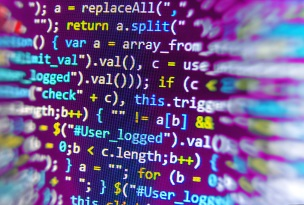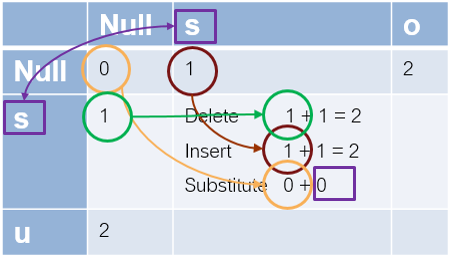## Loren on the Art of MATLABTurn ideas into MATLAB

Note

Loren on the Art of MATLAB has been archived and will not be updated.

# 40-year-old Algorithm That Cannot Be Improved

It is interesting to see how an obscure algorithm sometimes gets a break in news headlines like For 40 years, computer scientists looked for a solution that doesn’t exist in the Boston Globe. Today's guest blogger, Toshi Takeuchi, introduces us to this curious computer algorithm.### Contents

#### Edit Distance

The Boston Globe article talks about an algorithm known as edit distance . This is actually an important algorithm used in natural language processing and computational biology for such applications as spell checking, OCR, and genomic sequencing. But this discovery is perhaps most interesting to computational biologists because the computational intensity grows in quadratic time with respect to the length of strings, and the human genome contains about 3 billion base pairs (and quadratic time of that to compute)! So it would have been enormously useful if there were faster alternatives.

Too bad there isn't a faster algorithm, but the article says this has been received with "something like relief among computer scientists, who can now stop beating their heads against a wall in search of a faster method that doesn't exist."

Are you ready to see how this fastest possible algorithm works?

#### Examples

It is a distance measure between a pair of strings by the minimum number of edit operations required to transform one into another, as a way to quantify how similar or dissimilar they are.

For example, 'string' can be transformed into 'bring' by two edit operations – delete 's', and substitute 'b' for 't'.

s t r i n g
* b r i n g

If you count each edit as 1, then this pair has the edit distance of 2.

Edit distances comes in different variants, but let's try this with the editdist function I created based on pseudocode I found on Wikipedia.

str1 = 'string';
str2 = 'bring';
editdist(str1, str2)

ans =
2


You can see this can be useful to determine the closest proper spelling of a word for spell correction or in a noisy scan using OCR.

Now let's try this for DNA sequence analysis to count the number of mutations, which are essentially 'typos'.

str1 = 'ACAAGATGCCATTGTCCACCGGCCTCCTGCCTGCTGCTCTCGGCCACCGCTGCCCTGCC';
str2 = 'ACCTGGAGGGTTTGTCCACCGGCCTCCTGCCTGCTCTCTCCGGCCACCGCTGCCCTGCC';
editdist(str1, str2)

ans =
9


#### The Algorithm

Let's go back to the first example to see how the algorithm works. There are three edit operations you consider: insert, delete or substitute. You have seen the delete and substitute. If you want to go from 'bring' to 'string' instead, then you would have to insert 's'. The algorithm must search for a minimum effort path through a sequence of possible edits from the first character to the last, and this could be a huge search space!

The solution to this issue is a dynamic programming approach that uses, of course, a matrix! Let's use an example from the Wagner–Fischer algorithm Wikipedia page, which also provides the pseudocode I mentioned earlier.

In this example, I will reproduce the example matrix from Wikipedia using table in MATLAB. I substitute 'a' in 'saturday' with 'o' because you cannot repeat the same letter as row names or variable names in tables.

str1 = 'sunday';
str2 = 'soturday';
[~, tbl, rows, cols] = editdist(str1, str2);
disp(array2table(tbl, 'RowNames', rows, 'VariableNames', cols))

            Null    s    o    t    u    r    d    a    y
____    _    _    _    _    _    _    _    _
Null    0       1    2    3    4    5    6    7    8
s       1       0    1    2    3    4    5    6    7
u       2       1    1    2    2    3    4    5    6
n       3       2    2    2    3    3    4    5    6
d       4       3    3    3    3    4    3    4    5
a       5       4    4    4    4    4    4    3    4
y       6       5    5    5    5    5    5    4    3


The first row or column represents a null string where each character is empty. You see 1 in the 's' row and 'Null' column because it represents a deletion of letter 's' in order to make it an empty character, and that counts as 1 edit operation, and 'u' row and 'Null' column is 2 because it is another deletion and now we have done 2 edits to turn 'su' to '**' if you consider '*' to be an empty character. So the first column and row increases from 0 to the length of the string because we are just adding up the number of delete (column) or insert (row) operations.

Now let's work on the rest of the matrix. For each element in the matrix, you just look at the 3 neighbor elements, add 1 to their values, and use the minimum of the three for the given element.

• the element to the left = previous cost for a delete operation + 1 ... this is the current deletion cost
• the element above = previous cost for an insert operation + 1 ... this is the current insertion cost
• the element to the upper left = previous cost for a substitute operation + 1 if the letters differ, or + 0 if the letters are the same ... this is the current substitution cost

Let's work out the case of going from 's' to 's'.

• the element to the left is 1, so the current delete cost = 1+1 =2
• the one above is also 1, so the current insertion cost = 1+1 =2
• the one to the upper left is 0, and we are not changing the letter, so the current substitution cost = 0 + 0 = 0. This is also the minimum of the three, so this is 0.Now, from 's' to 'so', delete is 3, insert is 1, and substitute is 2, so it is 1. You continue filling out the matrix and the value you have in the last element is the minimum edit distance.

If you insert 'o' and 't', and substitute 'n' with 'r', then 'sunday' is transformed to 'soturday' with a minimum of three edit operations, so this matches with the computed result.

s * * u n d a y
s o t u r d a y

Check out my editdist function that implements this algorithm.

We happen to use edit distance at a character level, but we can use it on a string level, say, to compare two sentences generated by machine translation or a speech recognition system, or perhaps to detect plagiarism.

edit distance is a way of quantifying how dissimilar two strings
edit distance is a string metric for measuring the difference between two sequences.

Try modifying editdist to get it work on a string level and share your experience here!

Published with MATLAB® R2015b